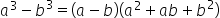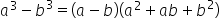Maths-
General
Easy

Question

# Prove the difference of cubes identity :Hint:

## The correct answer is: Hence, the proof

### Step 1 of 2:The given equation isThis can be proved by multiplying the RHS of the equation and simplifying to form the LHS.Step 2 of 2:Multiply the RHS in the given equation,Hence, the proof.

To prove an equation to be equal, we have to prove either the RHS is equal to the LHS or vice versa. The side should be selected based on the simplification process.#### With Turito Foundation.#### Get an Expert Advice From Turito.